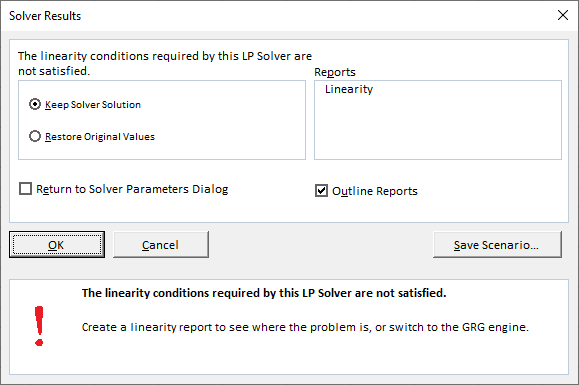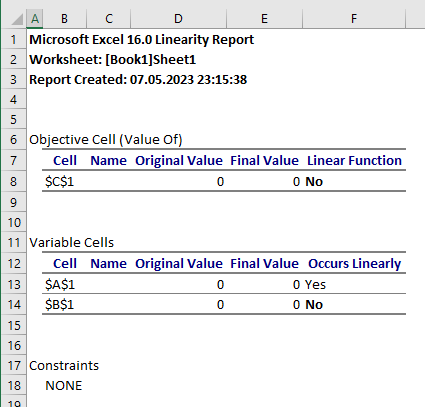# Librecalc solver cant do products

I put C1 to be =PRODUCT(A1:B1)
I called tools->solver
had it find C1 with value 6
I had it change A1:B1 cells
constraints were: 1. A1:B1 <= 4 and 2. A1:B1 >= 1
it either returns 0 or 1 for A1 and B1 or simply refuses to run, saying constraint is wrong(this happens when I add a constraint saying C1 = 6).
solver runs fine when I set C1 to =SUM(A1:B1)

Compare with:

I put X to cell A1, Y to cell B1, …, then select cells …, then call Tools->Solver, make sure that target cell is …, the other settings in the dialog are … . When I press Solve, I expect “this”, but instead, I get “that”.

Guess which description would allow people to see the problem easier.

problem is that tools->solver does not run when optimizing a cell containing product formula but works fine when the cell contains sum formula.
how do I make solver work well with cells containing product formula.

Reproducible.
This is a bug. The solver does not consider the constraints when doing some preparational calculations (it puts 0, 1, and 2 to cells unconditionally, and at each iteration, all cells except one are 0). This results in rejecting the model (it gets no change in result, no matter how each cell is modified independently).

The model can be modified: put `=PRODUCT(A1+1;B1+1)` to `C1`. But this would result in a strange result “10”, with the variables being 4 and 1. It seems it doesn’t consider how can a change to the second argument affect the result.

In essence: file a bug report.

On the other hand:
Excel’s Solver plugin, using their Simplex LP solver, also refuses this model. It shows this dialog:and the report looks likeSo maybe this is just an inappropriate model for linear solvers in general?

I solved my problem by using another method that was linear.
apparently x*y results in a non-linear system of equations which these linear solvers refuse to work with.